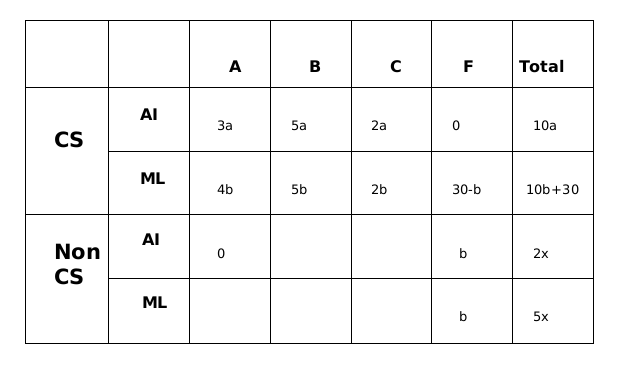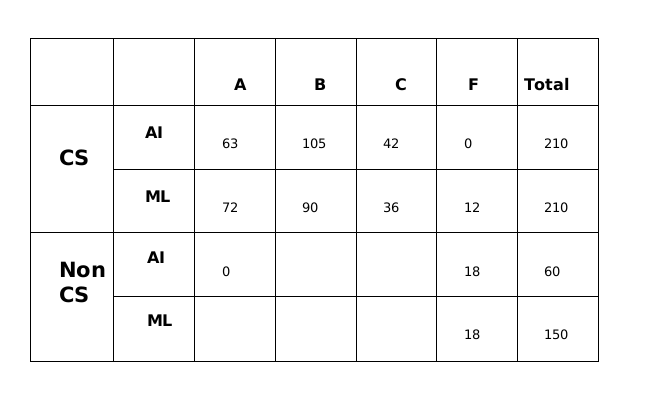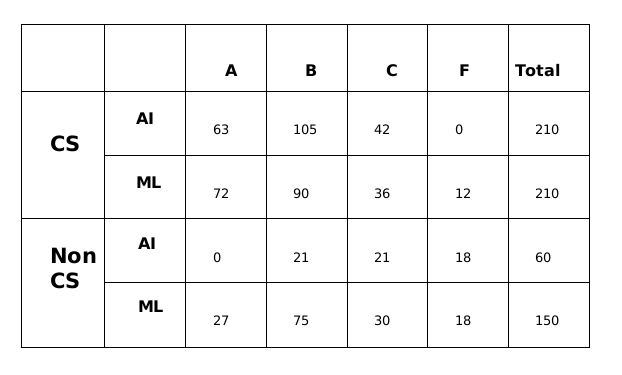### CAT 2022 Question Paper (Slot 3) Question 36

Instructions

All the first-year students in the computer science (CS) department in a university take both the courses (i) AI and (ii) ML. Students from other departments (non-CS students) can also take one of these two courses, but not both. Students who fail in a course get an F grade;others pass and are awarded A or B or C grades depending on their performance. The following are some additional facts about the number of students who took these two courses this year and the grades they obtained.

1.The numbers of non-CS students who took AI and ML were in the ratio 2 : 5.
2.The number of non-CS students who took either AI or ML was equal to the number of CS students.
3.The numbers of non-CS students who failed in the two courses were the same and their total is equal to the number of CS students who got a C grade in ML.
4. In both the courses, 50% of the students who passed got a B grade. But, while the numbers of students who got A and C grades were the same for AI, they were in the ratio 3 :2 for ML.
5. No CS student failed in AI, while no non-CS student got an A grade in AI.
6.The numbers of CS students who got A, B and C grades respectively in AI were in the ratio 3 : 5 : 2, while in ML the ratio was 4 : 5 : 2.
7.The ratio of the total number of non-CS students failing in one of the two courses to the  number of CS students failing in one of the two courses was 3 : 1.
8. 30 students failed in ML.

Question 36

# How many CS students failed in ML?

Solution

Let the number of students in non-CS be 7x so no.of students taking AI in non-CS is 2x and no. of students taking ML in non-CS is 5x

All the CS students have taken both the courses, we are given this in the first line of the set.

From statement 5, we can conclude that 0 students from CS failed in AI and 0 students from non-CS got grade A in AI.

From statement 6, let us say that the numbers of CS students who got A, B and C grades respectively in AI were in the ratio 3a, 5a and 2a, while in ML the ratio was 4b, 5b and 2b.

From statement 3 we can say that the number of non- CS students who failed in AI and ML are b in each category.

Now from statement 8, we can say that number of CS students who failed in MI is equal to 30-b.From statement 7, we can say that, $$\frac{2b}{30-b}=\frac{3}{1}$$

or, 2b = 90-3b

or, b= 18

CS students take both the AI and ML courses, therefore 10a= 10b +30

or, a= b+3 = 21

From statement 2, we can say that 10a = 7x

or, x = 30

Substituting the values of a, b and x in the above table.A total of 270 students took AI out of which 252 students passed and a total of 360 students took ML out of which 330 students passed.

From statement 4, we can say that out of the 252 students who passed in AI, 126 of them got Grade B and 63 got Grade A and 63 got Grade C.

Similarly, We can say that out of the 330 students who passed in ML, 165 of them got Grade C and 99 got Grade A and 66 got Grade C.

Therefore, the final table which we get is